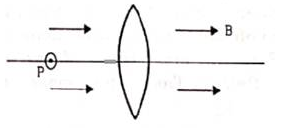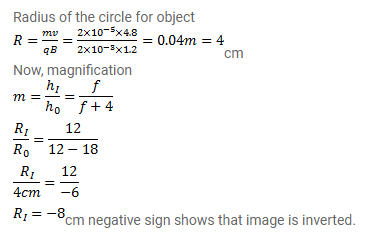# Figure shows a convex lens of focal length 12cm lying

Question:

Figure shows a convex lens of focal length $12 \mathrm{~cm}$ lying in a uniform magnetic field B of magnitude $1.2 \mathrm{~T}$ parallel to its principal axis. A particle having a charge $2.0 \times 10^{-3} \mathrm{C}$ and mass $2.0 \times 10^{-5} \mathrm{~kg}$ is projected perpendicular to the plane of the diagram with a speed of $4.8 \mathrm{~m} / \mathrm{s}$. The particle moves along a circle with its center on the principal axis at a distance of $18 \mathrm{~cm}$ from the lens. Show that the image of the particle goes along a circle and find the radius of that circle.Solution: